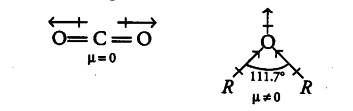# Explain why O = C = 0 is non-polar while R—O—R is polar?

CO _{ 2 } is a linear molecule. The dipole moment of two C = O bonds are equal and opposite. Hence, they cancel each other so, the dipole moment of CO _{ 2 } is zero and it is a non-polar molecule.While for ethers, two dipoles are pointing in the same direction. These two dipoles do not cancel the effect of each other. Therefore, there is a finite resultant dipoles and hence, R—O—R is a polar molecule.